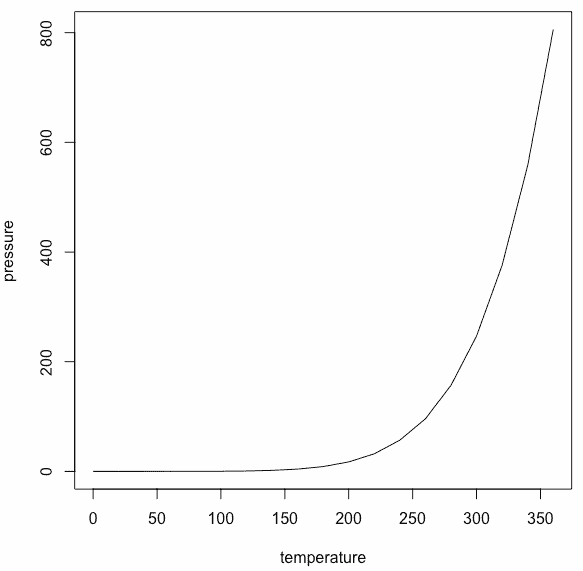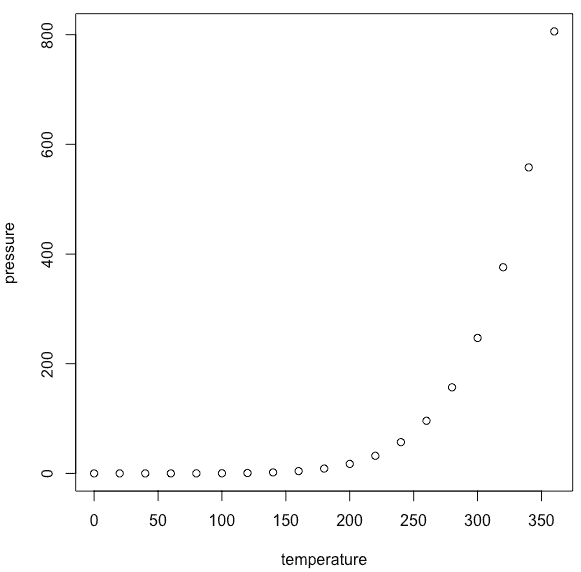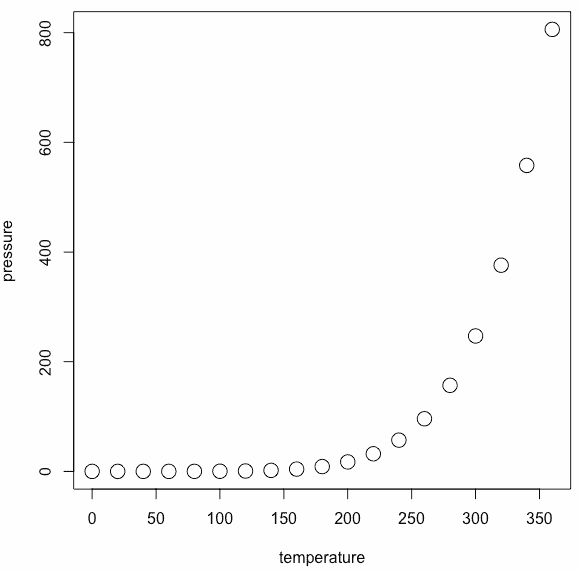# How to Create a Simple dataset Plot using the plot() Function

R provides many built-in datasets; for this example, we will use the pressure dataset. The pressure dataset contains observations of the vapor pressure of mercury over a range of temperatures.

``head(pressure)``

#### Output

``````   temperature    pressure
1      0            0.0002
2     20            0.0012
3     40            0.0060
4     60            0.0300
5     80            0.0900
6    100            0.2700``````

To create a dataset plot, use the plot() function.

``plot(pressure, type="l")``

#### OutputHere, we have plotted the line graph, but if you don’t pass type=”l,” it will create a point chart.

``plot(pressure)``

#### OutputTo modify the size of the plotted characters, use the cex (character expansion) argument.

``plot(pressure, cex = 2)``

#### OutputThat’s it.